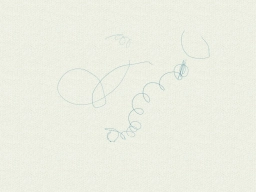# Miss Patel

Miss Patel thinks her class of 20 students will average 95 on a math test. Before she grades the last student's test, the class average is 97 . What is the lowest possible score on the 20 th test for the class to average 95?

x =  57

### Step-by-step explanation:Did you find an error or inaccuracy? Feel free to write us. Thank you!

Tips for related online calculators
Looking for help with calculating arithmetic mean?
Looking for a statistical calculator?
Do you have a linear equation or system of equations and looking for its solution? Or do you have a quadratic equation?
Do you want to round the number?

#### Grade of the word problem:

We encourage you to watch this tutorial video on this math problem: